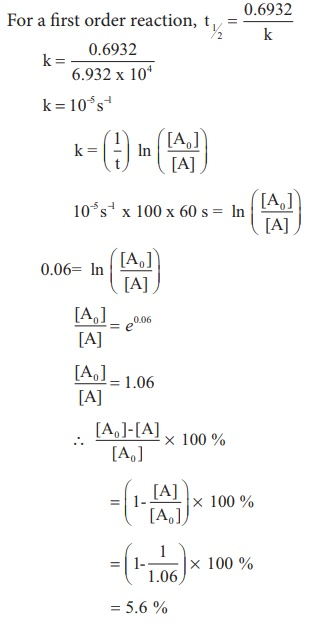Home | | Chemistry 12th Std | Half life period of a reaction

# Half life period of a reaction

The half life of a reaction is defined as the time required for the reactant concentration to reach one half its initial value. For a first order reaction, the half life is a constant i.e., it does not depend on the initial concentration.

Half life period of a reaction:

The half life of a reaction is defined as the time required for the reactant concentration to reach one half its initial value. For a first order reaction, the half life is a constant i.e., it does not depend on the initial concentration.

The rate constant for a first order reaction is given byLet us calculate the half life period for a zero order reaction.Hence, in contrast to the half life of a first order reaction, the half life of a zero order reaction is directly proportional to the initial concentration of the reactant.

Example 4

A first order reaction takes 8 hours for 90% completion. Calculate the time required for 80% completion. (log 5 = 0.6989 ; log10 = 1)

Solution:

For a first order reaction,Let [A0 ] = 100M

When

t = t90% ; [A]=10M (given that t90 % =8hours)

t = t80% ; [A]=20MFind the value of k using the given dataSubstitute the value of k in equation (2)t80% = 8hours x 0.6989

t80% = 5.59hours

Example 5

(ii) The half life of a first order reaction x → products is 6.932 x 104s at 500K . What percentage of x would be decomposed on heating at 500K for 100 min. (e0.06 = 1.06)

Solution:

Given t1/2 = 0.6932 x 104 s

To solve :2 when t=100 min,

[ [A0 ] −[A] / [A0]  ] x 100 = ?

We know that

For a first order reaction, t1/2 = 0.6932 /  kk = 10−5 s−1

Example 6

Show that in case of first order reaction, the time required for 99.9% completion is nearly ten times the time required for half completion of the reaction.Evaluate yourself:

1. In a first order reaction A → products 60% of the given sample of A decomposes in 40 min. what is the half life of the reaction?

2. The rate constant for a first order reaction is 2.3 X 10 −4 s−1 If the initial concentration of the reactant is 0.01M . What concentration will remain after 1 hour?

3. Hydrolysis of an ester in an aqueous solution was studied by titrating the liberated carboxylic acid against sodium hydroxide solution. The concentrations of the ester at different time intervals are given below.Show that, the reaction follows first order kinetics.

Tags : Chemical Kinetics , 12th Chemistry : UNIT 7 : Chemical Kinetics
Study Material, Lecturing Notes, Assignment, Reference, Wiki description explanation, brief detail
12th Chemistry : UNIT 7 : Chemical Kinetics : Half life period of a reaction | Chemical Kinetics